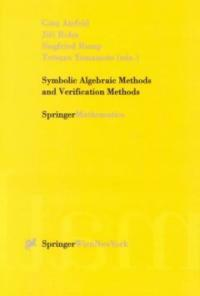> 상세정보

# 상세정보## Symbolic algebraic methods and verification methods

자료유형
단행본
개인저자
Alefeld, G. , 1941-
서명 / 저자사항
Symbolic algebraic methods and verification methods / Goetz Alefeld ... [et al.] (eds.).
발행사항
Wien ;   New York :   Springer ,   c2001.
형태사항
ix, 266 p. : ill. ; 25 cm.
총서사항
SpringerMathematics
ISBN
3211835938 (pbk. : alk. paper) 9783211835937 (pbk. : alk. paper)
서지주기
Includes bibliographical references.
일반주제명
Computer arithmetic. Floating-point arithmetic. Numerical calculations -- Verification.
 000 01049camuu22003134a 4500 001 000045517301 005 20090420151611 008 001122s2001 au a b 000 0 eng 010 ▼a 00067105 020 ▼a 3211835938 (pbk. : alk. paper) 020 ▼a 9783211835937 (pbk. : alk. paper) 035 ▼a (KERIS)REF000006168688 040 ▼a DLC ▼c DLC ▼d DLC ▼d 211009 042 ▼a pcc 050 0 0 ▼a QA76.9.M35 ▼b S92 2001 082 0 4 ▼a 004/.01/512 ▼2 22 090 ▼a 004.01512 ▼b S986 245 0 0 ▼a Symbolic algebraic methods and verification methods / ▼c Goetz Alefeld ... [et al.] (eds.). 260 ▼a Wien ; ▼a New York : ▼b Springer , ▼c c2001. 300 ▼a ix, 266 p. : ▼b ill. ; ▼c 25 cm. 490 1 ▼a SpringerMathematics 504 ▼a Includes bibliographical references. 650 0 ▼a Computer arithmetic. 650 0 ▼a Floating-point arithmetic. 650 0 ▼a Numerical calculations ▼x Verification. 700 1 ▼a Alefeld, G. , ▼d 1941- 830 0 ▼a Springer mathematics. 945 ▼a KINS

### 소장정보

No. 소장처 청구기호 등록번호 도서상태 반납예정일 예약 서비스
No. 1 소장처 청구기호 004.01512 S986 등록번호 121183197 도서상태 대출가능 반납예정일 예약 서비스

### 컨텐츠정보

#### 목차

`Introduction (G. Alefeld, J. Rohn, S. Rump, T. Yamamoto).- Topological Concepts for Hierarchies of Variables, Types and Controls (R. Albrecht).- Modifications of the Oettli-Prager Theorem with Application to the Eigenvalue Problem (G. Alefeld, V. Kreinovich, G. Mayer).- Symbolic-Numeric Algorithms for Polynomials. Some Recent Results (R. Corless).- Symbolic-Numeric QD-Algorithms with Applications in Function Theory and Linear Algebra (A. Cuyt).- On the Isoefficiency of the Parallel Dscartes Method (Th. Decker, W. Krandick).- Matrix Methods for Solving Algebraic Systems (I. Z. Emiris).- A Feasibility Result for Interval Gaussian Elimination Relying on Graph Structure (A. Frommer).- Solution of Systems of Polynomial Equations by Using Bernstein Expansion (J. Garloff, A. P. Smith).- Symbolic-Algebraic Computations in Modeling Language for Mathematical Programming (D. M. Gay).- Translation of Taylor Series into LFT Expansions (R. Heckmann).- Quasi Convex-Concave Extensions (Chr. Jansson).- Rewriting, Induction and Decision Procedures: A Case Study of Presburger Arithmetic (D. Kapur).- Derivative-Based Subdivision in Multi-dimensional Verified Gaussian Quadrature (B. Lang).- On the Shape of the Fixed Points of [f]([x]) = [A][x] [b].- Exact Computation with leda_real - Theory and Geometric Applications (K. Mehlhorn, St. Schirra).- Numerical Verification Method for Solutions of Nonlinear Hyperbolic Equations (T. Minamoto).- Geometric Series Bounds for the Local Errors of Taylor Methods for Linear n-th-Order ODEs (M. Neher).- Save Numerical Error Bounds for Solutions of Nonlinear Elliptic Boundary Value Problems (M. Plum).- Fast Verification Algorithms in MATLAB (S. Rump).- The Linear Complementarity Problem with Interval Data (U. Schafer).- Some Numerical Methods for Nonlinear Least Squares Problems (St. Shakhno).- A New Insight of the Shortley-Weller Approximation for Dirichlet Problems (T. Yamamoto).- How Orthogonality is Lost in Krylov Methods (J. Zemke).`

### 관련분야 신착자료

#### 데이터 통신 = 3판

Forouzan, Behrouz A. (2022)

#### 컴퓨터 구조 및 설계 : 하드웨어/소프트웨어 인터페이스

Patterson, David A. (2022)

みやたひろし (2022)

#### Quantum computing : an applied approach / 2nd ed

Hidary, Jack D (2021)

왕종수 (2022)

성동수 (2022)

박양수 (2022)

강환수 (2022)

#### 디지털 트윈 개발 및 클라우드 배포 : Simulink, Simscape, AWS를 활용해 클라우드 기반 다이나믹 모델 개발하기

Khaled, Nassim (2022)

정영철 (2022)

#### 메타버스를 디자인하라 : XR이 지향하는 미래 공간 구현하기

Hillmann, Cornel (2022)

황민정 (2021)

#### 공간 웹 : 웹 3.0시대의 기술이 삶, 비즈니스, 사회에 미치는 영향

René, Gabriel (2021)

#### 1일 1로그 100일 완성 IT 지식 : 하드웨어, 소프트웨어, 통신, 데이터 4가지 IT 근육으로 디지털 문해력 기르기

Kernighan, Brian W. (2021)

문유진 (2021)

이근우 (2022)

김효곤 (2022)